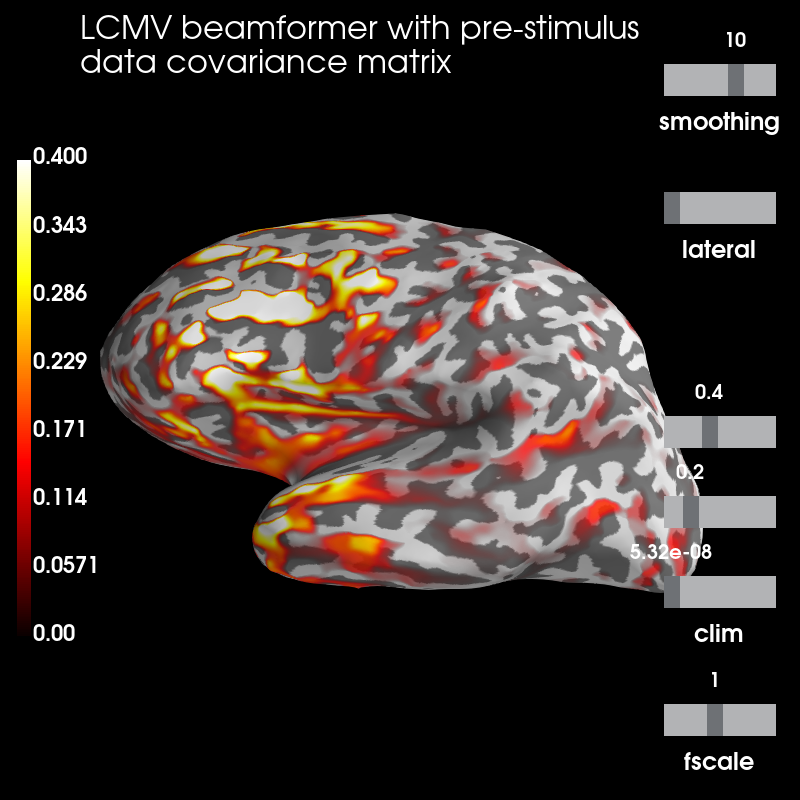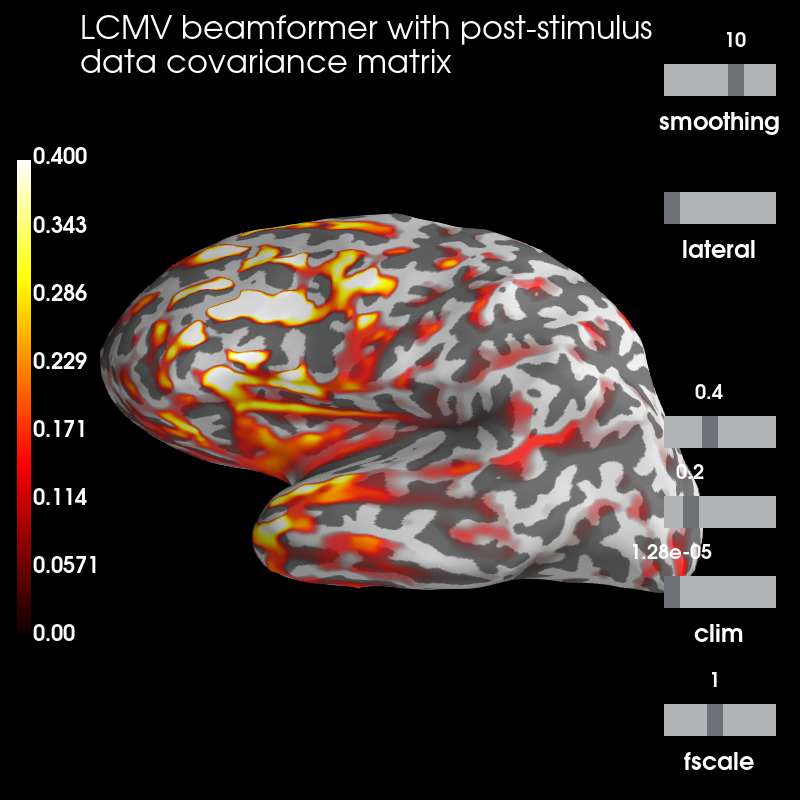# Compute cross-talk functions for LCMV beamformers¶

Visualise cross-talk functions at one vertex for LCMV beamformers computed with different data covariance matrices, which affects their cross-talk functions.

# Author: Olaf Hauk <olaf.hauk@mrc-cbu.cam.ac.uk>
#

import mne
from mne.datasets import sample
from mne.beamformer import make_lcmv, make_lcmv_resolution_matrix
from mne.minimum_norm import get_cross_talk

print(__doc__)

data_path = sample.data_path()
subjects_dir = data_path + '/subjects/'
fname_fwd = data_path + '/MEG/sample/sample_audvis-meg-eeg-oct-6-fwd.fif'
fname_cov = data_path + '/MEG/sample/sample_audvis-cov.fif'
fname_evo = data_path + '/MEG/sample/sample_audvis-ave.fif'
raw_fname = data_path + '/MEG/sample/sample_audvis_filt-0-40_raw.fif'

# only pick good EEG/MEG sensors
picks = mne.pick_types(raw.info, meg=True, eeg=True, exclude='bads')

# Find events
events = mne.find_events(raw)

# event_id = {'aud/l': 1, 'aud/r': 2, 'vis/l': 3, 'vis/r': 4}
event_id = {'vis/l': 3, 'vis/r': 4}

tmin, tmax = -.2, .25  # epoch duration
epochs = mne.Epochs(raw, events, event_id=event_id, tmin=tmin, tmax=tmax,

# covariance matrix for pre-stimulus interval
tmin, tmax = -.2, 0.
cov_pre = mne.compute_covariance(epochs, tmin=tmin, tmax=tmax,
method='empirical')

# covariance matrix for post-stimulus interval (around main evoked responses)
tmin, tmax = 0.05, .25
cov_post = mne.compute_covariance(epochs, tmin=tmin, tmax=tmax,
method='empirical')

# use forward operator with fixed source orientations
forward = mne.convert_forward_solution(forward, surf_ori=True,
force_fixed=True)

# get valid measurement info
info = raw.info

# regularize noise covariance (we used 'empirical' above)
noise_cov = mne.cov.regularize(noise_cov, info, mag=0.1, grad=0.1,
eeg=0.1, rank='info')


Out:

Opening raw data file /home/circleci/mne_data/MNE-sample-data/MEG/sample/sample_audvis_filt-0-40_raw.fif...
Read a total of 4 projection items:
PCA-v1 (1 x 102)  idle
PCA-v2 (1 x 102)  idle
PCA-v3 (1 x 102)  idle
Average EEG reference (1 x 60)  idle
Range : 6450 ... 48149 =     42.956 ...   320.665 secs
Reading 0 ... 41699  =      0.000 ...   277.709 secs...
319 events found
Event IDs: [ 1  2  3  4  5 32]
143 matching events found
Applying baseline correction (mode: mean)
Created an SSP operator (subspace dimension = 4)
4 projection items activated
Computing rank from data with rank=None
Using tolerance 4.9e-09 (2.2e-16 eps * 305 dim * 7.3e+04  max singular value)
Estimated rank (mag + grad): 302
MEG: rank 302 computed from 305 data channels with 3 projectors
Using tolerance 2.8e-11 (2.2e-16 eps * 59 dim * 2.1e+03  max singular value)
Estimated rank (eeg): 58
EEG: rank 58 computed from 59 data channels with 1 projector
Created an SSP operator (subspace dimension = 4)
Setting small MEG eigenvalues to zero (without PCA)
Setting small EEG eigenvalues to zero (without PCA)
Reducing data rank from 364 -> 360
Estimating covariance using EMPIRICAL
Done.
Number of samples used : 4433
[done]
Computing rank from data with rank=None
Using tolerance 5.9e-09 (2.2e-16 eps * 305 dim * 8.7e+04  max singular value)
Estimated rank (mag + grad): 302
MEG: rank 302 computed from 305 data channels with 3 projectors
Using tolerance 3.8e-11 (2.2e-16 eps * 59 dim * 2.9e+03  max singular value)
Estimated rank (eeg): 58
EEG: rank 58 computed from 59 data channels with 1 projector
Created an SSP operator (subspace dimension = 4)
Setting small MEG eigenvalues to zero (without PCA)
Setting small EEG eigenvalues to zero (without PCA)
Reducing data rank from 364 -> 360
Estimating covariance using EMPIRICAL
Done.
Number of samples used : 4433
[done]
Computing patch statistics...
[done]
Computing patch statistics...
[done]
Desired named matrix (kind = 3523) not available
Read MEG forward solution (7498 sources, 306 channels, free orientations)
Desired named matrix (kind = 3523) not available
Read EEG forward solution (7498 sources, 60 channels, free orientations)
MEG and EEG forward solutions combined
Source spaces transformed to the forward solution coordinate frame
Average patch normals will be employed in the rotation to the local surface coordinates....
Converting to surface-based source orientations...
[done]
366 x 366 full covariance (kind = 1) found.
Read a total of 4 projection items:
PCA-v1 (1 x 102) active
PCA-v2 (1 x 102) active
PCA-v3 (1 x 102) active
Average EEG reference (1 x 60) active
Computing rank from covariance with rank='info'
MEG: rank 302 after 3 projectors applied to 305 channels
EEG: rank 58 after 1 projector applied to 59 channels
8 projection items activated
MAG regularization : 0.1
Created an SSP operator (subspace dimension = 3)
Computing rank from covariance with rank={'meg': 302, 'eeg': 58}
Using tolerance 2.5e-14 (2.2e-16 eps * 102 dim * 1.1  max singular value)
Estimated rank (mag): 99
MAG: rank 99 computed from 102 data channels with 3 projectors
Setting small MAG eigenvalues to zero (without PCA)
Computing rank from covariance with rank={'meg': 302, 'eeg': 58, 'mag': 99}
Using tolerance 1.8e-13 (2.2e-16 eps * 203 dim * 3.9  max singular value)
GRAD: rank 203 computed from 203 data channels with 0 projectors
Setting small GRAD eigenvalues to zero (without PCA)
EEG regularization : 0.1
Created an SSP operator (subspace dimension = 1)
Computing rank from covariance with rank={'meg': 302, 'eeg': 58, 'mag': 99, 'grad': 203}
Setting small EEG eigenvalues to zero (without PCA)


## Compute LCMV filters with different data covariance matrices¶

# compute LCMV beamformer filters for pre-stimulus interval
filters_pre = make_lcmv(info, forward, cov_pre, reg=0.05,
noise_cov=noise_cov,
pick_ori=None, rank=None,
weight_norm=None,
reduce_rank=False,
verbose=False)

# compute LCMV beamformer filters for post-stimulus interval
filters_post = make_lcmv(info, forward, cov_post, reg=0.05,
noise_cov=noise_cov,
pick_ori=None, rank=None,
weight_norm=None,
reduce_rank=False,
verbose=False)


## Compute resolution matrices for the two LCMV beamformers¶

rm_pre = make_lcmv_resolution_matrix(filters_pre, forward, info)

rm_post = make_lcmv_resolution_matrix(filters_post, forward, info)

# compute cross-talk functions (CTFs) for one target vertex
sources = 

stc_pre = get_cross_talk(rm_pre, forward['src'], sources, norm=True)

stc_post = get_cross_talk(rm_post, forward['src'], sources, norm=True)


Out:

    364 out of 366 channels remain after picking
Dimensions of LCMV resolution matrix: 7498 by 7498.
364 out of 366 channels remain after picking
Dimensions of LCMV resolution matrix: 7498 by 7498.


## Visualise¶

vertno_lh = forward['src']['vertno']  # vertex of selected source
verttrue = [vertno_lh[sources]]  # pick one vertex

brain_pre = stc_pre.plot('sample', 'inflated', 'lh', subjects_dir=subjects_dir,
figure=1, clim=dict(kind='value', lims=(0, .2, .4)))

brain_pre.add_text(0.1, 0.9, 'LCMV beamformer with pre-stimulus\ndata '
'covariance matrix', 'title', font_size=16)

brain_post = stc_post.plot('sample', 'inflated', 'lh',
subjects_dir=subjects_dir,
figure=2, clim=dict(kind='value', lims=(0, .2, .4)))

brain_post.add_text(0.1, 0.9, 'LCMV beamformer with post-stimulus\ndata '
'covariance matrix', 'title', font_size=16)

# mark true source location for CTFs
color='green')